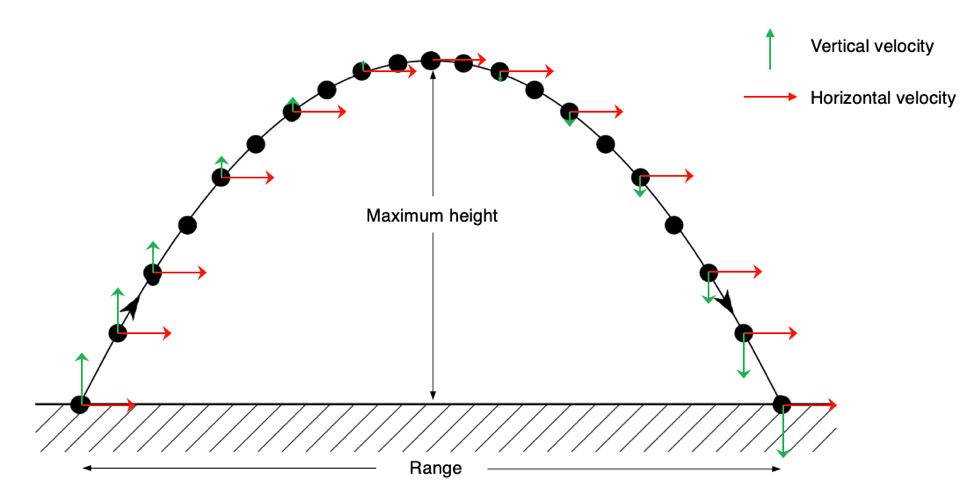# M5-S1: Introduction to Projectile Motion

• ### Analyse the motion of projectiles by resolving the motion into horizontal and vertical components, making the following assumptions:

• a constant vertical acceleration due to gravity
• zero air resistance

### Galileo’s Analysis of Projectile Motion

• Projectile motion can be analysed as a form of two-dimensional motion, consisting of a horizontal rectilinear motion (forward-backward or left-right) and a vertical rectilinear motion (up-down).
• The path of projectile motion was first described by Galileo as parabolic and can be resolved into horizontal and vertical components:
• Horizontal component in the absence of zero resistance experience no acceleration
• Vertical component is affected by a constant acceleration due to gravity: 9.8 ms-2. This acceleration (which is a vector quantity) always acts downwards, towards the centre of Earth.
• These ‘components’ are horizontal and vertical velocities of an object in projectile motion. They are independent to one another, meaning changing one component does not affect the other.
• Horizontal velocity is always constant (no acceleration)
• Vertical velocity is not constant (affected by gravity)Diagram illustrates changes in horizontal (red) and vertical (green) velocities of an object in projectile motion. The maximum height and range (horizontal displacement) are also displayed.

In any projectile motion:

• Vertical velocity decreases in magnitude (vector shortens) when the projectile is travelling upwards, against gravity.
• Vertical velocity is zero at the tip (maximum height) of projectile motion.
• Vertical velocity changes direction and increases in magnitude (vector lengthens) when the projectile is travelling downwards, in the direction of gravity.
• The direction and magnitude of horizontal velocity remains constant.

### Assumptions

There are a few critical assumptions made in Galileo’s analysis of projectile motion:

• Air resistance will always be a factor during an object’s motion unless it occurs within a vacuum. Therefore, the horizontal velocity is usually not constant in real life.
• Gravitational acceleration (g) changes with altitude and the projectile’s location on Earth. (9.8 ms-1 is only an average value on the surface of Earth)